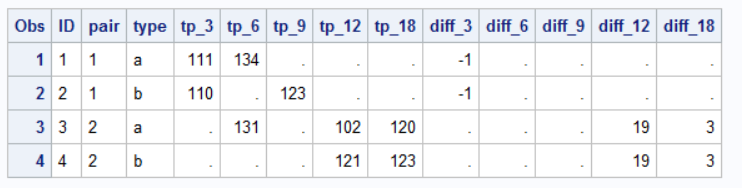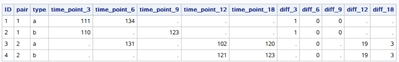## How will you calculate row differences within pairs for a set of variables?

This is the dataset

``````data dsin;
input ID\$ pair\$ type\$ time_point_3	time_point_6	time_point_9	time_point_12	time_point_18;
datalines;
1	1	a	111	134	.	.	.
2	1	b	110	.	123	.	.
3	2	a	.	131	.	102	120
4	2	b	.	.	.	121	123
;
run;``````

How will you calculate row differences within the same pair for each time_point? We want to get the following results:

``````data dsout;
input ID\$ pair\$ type\$ time_point_3	time_point_6	time_point_9	time_point_12	time_point_18 Diff_3	Diff_6	Diff_9	Diff_12	Diff_18;
datalines;
1	1	a	111	134	.	.	.	1		.	.	.
2	1	b	110	.	123	.	.	1		.	.	.
3	2	a	.	131	.	102	120	.	.	.	19	3
4	2	b	.	.	.	121	123	.	.	.	19	3
;
run;``````
1 ACCEPTED SOLUTION

Accepted Solutions

## Re: How will you calculate row differences within pairs for a set of variables?

If you are positive you always have exactly two observations per PAIR then you can use use DIF() function.  Use double DOW loop so only the second DIF() is kept and merged back onto the first observation.

``````data want;
do until(last.pair);
set dsin;
by pair;
diff_3=dif(tp_3);
diff_6=dif(tp_6);
diff_9=dif(tp_9);
diff_12=dif(tp_12);
diff_18=dif(tp_18);
end;
do until(last.pair);
set dsin;
by pair;
output;
end;
run;
``````8 REPLIES 8

## Re: How will you calculate row differences within pairs for a set of variables?

While you can do this with arrays, this type of data is easier to work with if you transpose to a LONG format.  I would consider something like:

``````proc transpose data=dsin out=vert (rename=(_name_=timepoint col1=value));
var time_point_3	time_point_6	time_point_9	time_point_12	time_point_18 ;
by id pair type ;
run ;

proc sort data=vert ;
by pair timepoint type ;
run ;

data want ;
set vert ;
by pair timepoint ;
Diff=dif(value) ;
if first.timepoint then call missing(diff) ;
run ;

proc print data=want ;
run ;``````

Note that returns -1 for the first difference, not 1.  You might be intending absolute value of the difference?

Check out the Boston Area SAS Users Group (BASUG) video archives: https://www.basug.org/videos.

Thank you!

## Re: How will you calculate row differences within pairs for a set of variables?

If you are positive you always have exactly two observations per PAIR then you can use use DIF() function.  Use double DOW loop so only the second DIF() is kept and merged back onto the first observation.

``````data want;
do until(last.pair);
set dsin;
by pair;
diff_3=dif(tp_3);
diff_6=dif(tp_6);
diff_9=dif(tp_9);
diff_12=dif(tp_12);
diff_18=dif(tp_18);
end;
do until(last.pair);
set dsin;
by pair;
output;
end;
run;
``````## Re: How will you calculate row differences within pairs for a set of variables?

Thank you! Yes, only two observations per pair.

## Re: How will you calculate row differences within pairs for a set of variables?

``````data want;
set dsin;
array old time_point_3	time_point_6	time_point_9	time_point_12	time_point_18;
array new tp3 tp6 tp9 tp12 tp18;
array diff diff_3 diff_6 diff_9 diff_12 diff_18;
do over old;
new= lag(old);
if old ne . and new ne . then diff=old-new;
end;
drop tp:;
run;

proc sort data=want;by pair descending type;run;

data want1;
set want;
by pair descending type;
array temp ;
array diff  diff_3--diff_18;
do i= 1 to 5;
retain temp;
if first.pair then temp{i}=diff{i};
if diff{i} eq . then diff{i}=temp{i};
end;
drop temp: i;
proc print;run; ``````Ksharp
Super User

## Re: How will you calculate row differences within pairs for a set of variables?

``````data dsin;
input ID\$ pair\$ type\$ time_point_3	time_point_6	time_point_9	time_point_12	time_point_18;
datalines;
1	1	a	111	134	.	.	.
2	1	b	110	.	123	.	.
3	2	a	.	131	.	102	120
4	2	b	.	.	.	121	123
;
run;
proc sql;
select *,
range(time_point_3) as diff_3,
range(time_point_6) as diff_6,
range(time_point_9) as diff_9,
range(time_point_12) as diff_12,
range(time_point_18) as diff_18
from dsin
group by pair;
quit;``````## Re: How will you calculate row differences within pairs for a set of variables?

Hi @Ksharp ,
What an efficient way to solve the problem!
Does this always return a positive value? Or is there a way(optional arguments) to control the result, like difference of first.variable from the last or vice versa...Ksharp
Super User

## Re: How will you calculate row differences within pairs for a set of variables?

"Does this always return a positive value?"
Yes . RANGE() always return positive value ,since it = max-min .

"Or is there a way(optional arguments) to control the result, like difference of first.variable from the last or vice versa... "
No, there is no other option, but I think you could use the skill posed by @Tom via LAG() function.
Discussion stats
• 8 replies
• 278 views
• 4 likes
• 5 in conversation